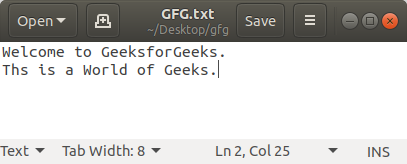Open In App
Related Articles

# Python Program to Reverse the Content of a File using Stack

Given a file, the task is to change the content in reverse order using Stack, as well as store the lines of that file in reverse order in Python.

Examples:

```Input:
1
2
3
4
5
Output:
5
4
3
2
1
```

### Approach to Python Program to Reverse a Stack Using Recursion

• Create an empty stack.
• One by one push every line of the file to the stack.
• One by one pop each line from the stack and put them back to the file.

Input File:### Code Explanation

Here first we create a stack class and then initialize an array in the class providing and provide the method to an array that follows the LIFO rule of the Python stack. The LIFO rule stands for Last Input Frist Output which is the basic rule of the stack after that we use that stack to store the data of the file and after storing the data we extract the data from the stack and print the data we append in the stack will come out first and like that we get the output data of the file reversed.

## Python3

 `# Creating Stack class (LIFO rule)``class` `Stack:` `    ``def` `__init__(``self``):` `        ``# Creating an empty stack``        ``self``._arr ``=` `[]` `    ``# Creating push() method.``    ``def` `push(``self``, val):``        ``self``._arr.append(val)` `    ``def` `is_empty(``self``):` `        ``# Returns True if empty``        ``return` `len``(``self``._arr) ``=``=` `0` `    ``# Creating Pop method.``    ``def` `pop(``self``):` `        ``if` `self``.is_empty():``            ``print``(``"Stack is empty"``)``            ``return` `        ``return` `self``._arr.pop()` `# Creating a function which will reverse``# the lines of a file and Overwrites the``# given file with its contents line-by-line``# reversed`  `def` `reverse_file(filename):` `    ``S ``=` `Stack()``    ``original ``=` `open``(filename)` `    ``for` `line ``in` `original:``        ``S.push(line.rstrip(``"\n"``))` `    ``original.close()` `    ``output ``=` `open``(filename, ``'w'``)` `    ``while` `not` `S.is_empty():``        ``output.write(S.pop()``+``";\n"``)` `    ``output.close()`  `# Driver Code``filename ``=` `"GFG.txt"` `# Calling the reverse_file function``reverse_file(filename)` `# Now reading the content of the file``with ``open``(filename) as ``file``:``    ``for` `f ``in` `file``.readlines():``        ``print``(f, end``=``"")`

Output:

```This is a World of Geeks.
Welcome to GeeksforGeeks.
```

Time complexity: O(n), where n is the number of lines in the file.
Auxiliary space: O(n), where n is the number of lines in the file.